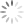# Cmd Markdown 简明语法手册

### 2. 分级标题

这是一个一级标题
============================

--------------------------------------------------

### 这是一个三级标题


• 无序列表项 一
• 无序列表项 二
• 无序列表项 三

1. 有序列表项 一
2. 有序列表项 二
3. 有序列表项 三

### 8. 代码块

这是一个代码块，此行左侧有四个不可见的空格。


### 9. 插入图像# Cmd Markdown 高阶语法手册

[TOC]

### 2. 标签分类

Tags： 数学 英语 Markdown

$表示行内公式： 质能守恒方程可以用一个很简洁的方程式$E=mc^2$来表达。 $$表示整行公式：$$\sum_{i=1}^n a_i=0f(x_1,x_x,\ldots,x_n) = x_1^2 + x_2^2 + \cdots + x_n^2 \sum^{j-1}{k=0}{\widehat{\gamma}{kj} z_k}$$访问 MathJax 参考更多使用方法。 ### 6. 加强的代码块 支持四十一种编程语言的语法高亮的显示，行号显示。 非代码示例： $ sudo apt-get install vim-gnome


Python 示例：

@requires_authorization
def somefunc(param1='', param2=0):
'''A docstring'''
if param1 > param2: # interesting
print 'Greater'
return (param2 - param1 + 1) or None

class SomeClass:
pass

>>> message = '''interpreter
... prompt'''


JavaScript 示例：

/**
* nth element in the fibonacci series.
* @param n >= 0
* @return the nth element, >= 0.
*/
function fib(n) {
var a = 1, b = 1;
var tmp;
while (--n >= 0) {
tmp = a;
a += b;
b = tmp;
}
return a;
}

document.write(fib(10));


### 7. 流程图

#### 示例

st=>start: Start:>https://www.zybuluo.com
io=>inputoutput: verification
cond=>condition: Yes or No?
e=>end

st->io->op->cond
cond(yes)->e
cond(no)->sub->io


### 8. 序列图

#### 示例 1

Alice->Bob: Hello Bob, how are you?
Note right of Bob: Bob thinks
Bob-->Alice: I am good thanks!


#### 示例 2

Title: Here is a title
A->B: Normal line
B-->C: Dashed line
C->>D: Open arrow
D-->>A: Dashed open arrow


### 9. 甘特图

    title 项目开发流程
section 项目确定
需求分析       :a1, 2016-06-22, 3d
可行性报告     :after a1, 5d
概念验证       : 5d
section 项目实施
概要设计      :2016-07-05  , 5d
详细设计      :2016-07-08, 10d
编码          :2016-07-15, 10d
测试          :2016-07-22, 5d
section 发布验收
发布: 2d
验收: 3d


### 10. Mermaid 流程图

    A[Hard edge] -->|Link text| B(Round edge)
B --> C{Decision}
C -->|One| D[Result one]
C -->|Two| E[Result two]


### 11. Mermaid 序列图

    Alice->John: Hello John, how are you?
loop every minute
John-->Alice: Great!
end


### 13. 定义型列表

代码块（左侧有八个不可见的空格）


### 14. Html 标签

<table>
<tr>
<th rowspan="2">值班人员</th>
<th>星期一</th>
<th>星期二</th>
<th>星期三</th>
</tr>
<tr>
<td>李强</td>
<td>张明</td>
<td>王平</td>
</tr>
</table>


### 15. 内嵌图标

<i class="icon-weibo"></i>


<i class="icon-renren"></i>


### 16. 待办事宜 Todo 列表

- [ ] **Cmd Markdown 开发**
- [ ] 改进 Cmd 渲染算法，使用局部渲染技术提高渲染效率
- [ ] 支持以 PDF 格式导出文稿
- [x] 改进 LaTex 功能
- [x] 修复 LaTex 公式渲染问题
- [x] 新增 LaTex 公式编号功能 [语法参考](http://docs.mathjax.org/en/latest/tex.html#tex-eq-numbers)
- [ ] **七月旅行准备**
- [ ] 准备邮轮上需要携带的物品
- [ ] 浏览日本免税店的物品
- [x] 购买蓝宝石公主号七月一日的船票


• [ ] Cmd Markdown 开发
• [ ] 改进 Cmd 渲染算法，使用局部渲染技术提高渲染效率
• [ ] 支持以 PDF 格式导出文稿
• [x] 新增Todo列表功能 语法参考
• [x] 改进 LaTex 功能
• [x] 修复 LaTex 公式渲染问题
• [x] 新增 LaTex 公式编号功能 语法参考
• [ ] 七月旅行准备
• [ ] 准备邮轮上需要携带的物品
• [ ] 浏览日本免税店的物品
• [x] 购买蓝宝石公主号七月一日的船票

[^footnote]: 这是一个 注脚文本

[^footnote2]: 这是另一个 注脚文本